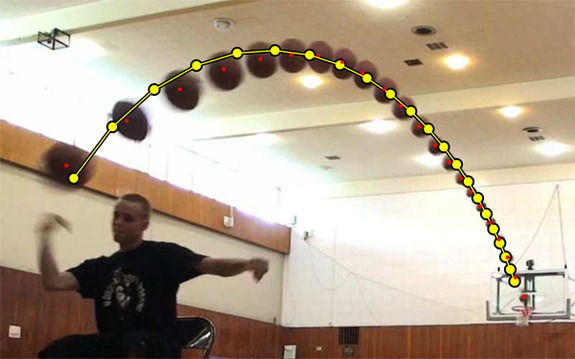top of page

## What is Force?

A force is a vector quantity capable of producing motion or a change in motion, which means that a force may result in a change in the velocity or acceleration. A force may act on an object, but the force may or may not cause a change in the motion of the object. If there are one or more opposing forces that cancel out an applied force, then no change in motion will occur (the net effect is zero; the forces are balanced). Therefore, a force does not necessarily produce a change in motion1. If there are no other forces, then the applied force on the object will produce a change in the velocity or acceleration of the object (the applied force is unbalanced). An example of the difference between balanced and unbalanced forces is shown below.

Image Source: Devanna Art

In the image above, we have balanced forces and unbalanced forces. The two ladies having a go at tug-of-war at the bottom right illustrate balanced forces. If both ladies were at 60 newtons of forces, they are balanced. Above that group is a team in which there is a shift in force as the magnitude has been increased by an additional 60 newtons on the right side of the equation. This is now an unbalanced force.bottom of page